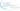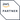# Dart vs JavaScript vs TypeScript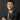Gordon Lau 劉偉中
2019-02-17## 應用範圍(Application)

React Native寫成的手機程式結構如下：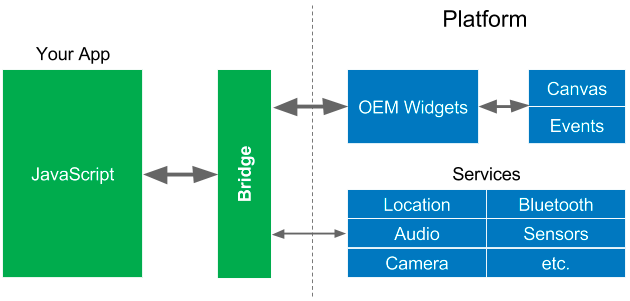Flutter寫成的手機程式結講則如下: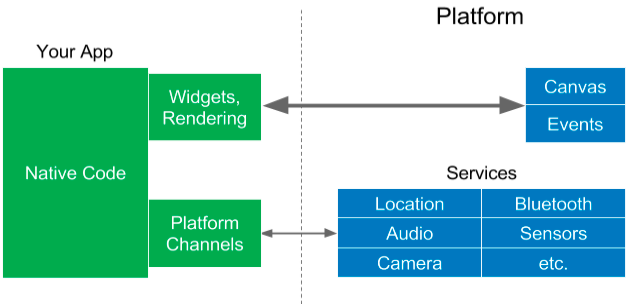Source

## 基本運行

JavaScript 和 TypeScript的例子都是：

`.css-qhxz92{max-width:100%;}`console.log("Hello World");``

Dart的例子：

``print("Hello World");``

``````node index.js
ts-node index.ts
dart main.dart``````

## 基本語法

### 變數(Variable)

JavaScript例子：

``````var num = 123;
let hello = "Hello Dart!";
const obj = { key1: 1234, key2: 5678};``````

TypeScript例子(例子中類別皆可省略):

``````var num:number = 123;
let hello:string = "Hello Dart!";
const obj:obj = { key1: 1234, key2: 5678};``````

Dart例子：

``````var num = 42;
dynamic hello = "Hello Dart!";
final obj = {"key1":1234,"key":5678};
hello = 1234;
String myName = "Tecky";
const PI = 3.141592654;
var x; // == null``````

JavaScript的例子與TypeScript除了額外的類別，幾乎一模一樣，反之Dart則非常不同，Dart有幾種不同的方法定義變數:

1. var: 定義一個新的變數，類別由數值所推論，所以如果將num重新賦值為String會出現Error，但賦值為同一類別則無問題。
2. dynamic: 定義一個新的變數，類別不定，重新賦值任何類別都無問題。
3. final: 定義一個新的常數，不可重新賦值。
4. 直接前置類別如String: 定義一個新的變數，類別由前置類別決定，如果將重新賦值為其他類別會出現Error，但賦值為同一類別則無問題
5. const: 與final類似，編譯常數，不可重新賦值。

### 運算符號(Operators)

JavaScript　及TypeScript例子：

``````let num = null;
if(!num){
console.log(`null is false`);
}
let zero = 0;
if(!zero){
console.log(`zero is false`);
}``````

``````var num = null;
if(num == null){
print(`null is not false`);
}
var zero = 0;
if(zero == 0){
print(`zero is not false`);
}``````

### 函數(Function)

JavaScript例子：

``````
function sum(a,b){
return a + b;
}``````

TypeScript例子(例子中回傳值的類別可省略):

``````function sum(a:number,b:number):number{
return a + b;
}``````

Dart例子：

``````sum(int a, int b){
return a+b;
}``````

Dart的例子中，如果不寫`int`的類別，那兩個參數都會是`dynamic` 類別，也就是任何類別都可以成為參數。

``````sum(a,b){
return a+b;
}
// equals to

sum(dynamic a,dynamic b){
return a+b;
}``````

``sum("1",1);``

``````main(){
sum(2,2);
}

sum(int a, int b){
return a+b;
}``````

## 進階語法

### 類別(Class)

``````import 'dart:math';

class Point{
num x;
num y;
num z= 0;

Point(num x, num y){
this.x = x;
this.y = y;
}

num distanceTo(Point other) {
var dx = x - other.x;
var dy = y - other.y;
return sqrt(dx * dx + dy * dy);
}
}

void main(){
var p1 = Point(2,2);
var p2 = new Point(4,5);
p1.distanceTo(p2);
}``````

``````class Point{

private x: number;
private y: number;
private z: number = 0;

constructor(x:number,y:number){
this.x = x;
this.y = y;
}

distanceTo(Point other){
let dx = x - other.x;
let dy = y - other.y;
return sqrt(dx * dx + dy * dy);
}
}

const p1 = new Point(2,2);
const p2 = new Point(4,5);
p1.distanceTo(p2);``````

### 非同步編程(Asynchronous Programming)

Dart的`async`與JavaScript幾乎一模一樣，分別只是先後次序寫法，以及必須寫下傳回值的類別，也就是`Future``Future`的概念與`JavaScript　Promise`無任何分別，只是名字上的分別。`Java`的非同步編程類別，也是稱為`Future`

``````Future main() async{
await someAsyncOperation();
}``````

``````async function main(){
await someAsyncOperation()
}``````

## 閱讀更多## 學術探討系列：型別推論(一) Type inference(I)Gordon Lau 劉偉中
2018-11-08## 到底React Hooks 有何特別？Gordon Lau 劉偉中
2018-11-27## 到底React Hooks有何特別（二）？淺談useEffect及useReducerGordon Lau 劉偉中
2018-11-29## Web Technology為何征服世界？Gordon Lau 劉偉中
2019-02-05

2007年，蘋果宣佈發佈第一代iPhone，標誌智能電話時代的開始;一年之後Android亦宣告面世，從此時起，智能電話的發展迅速，Ｍobile App成為軟件的代 名詞，筆者初初成為軟件工程師時，總有朋友詢問我是否正在開發Mobile App，縱使筆者的專業一直都是網頁及後端開發之上。而其時亦有不少預測，預測[網站將會被Mobile App完全取代](https://searchenginewatch.com/sew/opinion/2414336/the-final-hurdle-is-cleared-apps-will-replace-websites)。網站所用的HTML、CSS、JS等，亦將成為歷史，送入博物館之內。

##### 索取課程大綱
###### 提交後, 請檢查你的電郵